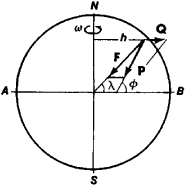# Gravity, Force of

The following article is from The Great Soviet Encyclopedia (1979). It might be outdated or ideologically biased.

## Gravity, Force of

the force P acting on any material particle located near the earth’s surface, where P is defined as the vector sum of the earth’s gravitational force F and the centrifugal force Q, which allows for the effect of the earth’s diurnal rotation (see Figure 1). The direction of the force of gravity is the direction of a plumb line—that is, of the vertical—at the given point of the earth’s surface, and the plane perpendicular to the plumb line is the horizontal plane. The angles λ and Φ determine the geocentric and astronomical latitudes, respectively.Figure 1

The quantity Q = mhω2 (where m is the mass of the particle, h the particle’s distance from the earth’s axis, and ω the angular velocity of the earth’s rotation) is very small compared with F because of the smallness of ω2. The force of gravity therefore differs little from the gravitational force. As an observer moves along the earth’s surface from one of the poles to the equator, the magnitude of the force of gravity decreases somewhat because of the increase in Q and because the earth is not a perfect sphere. The force of gravity is approximately 0.5 percent smaller at the equator than at the poles. The difference between the angles Φ and λ is also small; the greatest difference is about 11’ at λ = 45°. Under the action of the force of gravity a particle acquires an acceleration g = P/m. called the acceleration of gravity, which, like the force of gravity, varies with latitude.

At all points in a region whose dimensions are small compared with the earth’s radius, the force of gravity can be considered to have the same magnitude and direction—that is, it can be considered to form a uniform field of force. In this field the potential energy of a particle is Π = Pz, where z is the particle’s coordinate measured along the vertical upward from some initial level. When the particle moves from the position where z = z1, to the position where z = z2, the work done by the force of gravity is A = P(z1z2) and is independent of the particle’s trajectory and of the law governing the particle’s motion. The action of the force of gravity has a considerable influence on nearly all phenomena and processes occurring on the earth, both in nature (including animate nature) and in technology. (See alsoGRAVIMETRY.)

S. M. TARG

The Great Soviet Encyclopedia, 3rd Edition (1970-1979). © 2010 The Gale Group, Inc. All rights reserved.
Site: Follow: Share:
Open / Close